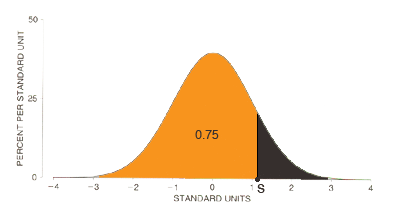SEARCH HOMEMath Central Quandaries & QueriesI am stuck on this question. I am not sure if using the z score will help me get the answer I need. Here is the question? The average length of time per week that students at this university spend on homework is normally distributed with a mean of 18 hours and a standard deviation of 3 hours. If Diane spends more time on homework each week than 75% of students, what is the minimum time she must spend? Z= 75-18 /3 z=19Hi Racquel,

You do need to use the Z score but you have applied it incorrectly. You know that the times that students spend on homework is normally distributed with a mean of 18 hours and a standard deviation of 3 hours. You don't know how many hours Diane spends so let's call it X hours. It is this value that you need to convert to a Z score using

Z = (X - 18)/3.

Now you have a standard normal random variable. In the diagram below you want the value S so that the area under the curve, to the left of S is 75% or 0.75.Use you normal table to find the value of S and the solve the equation

(X - 18)/3 = S

for X.

I hope this helps,
PennyMath Central is supported by the University of Regina and The Pacific Institute for the Mathematical Sciences.